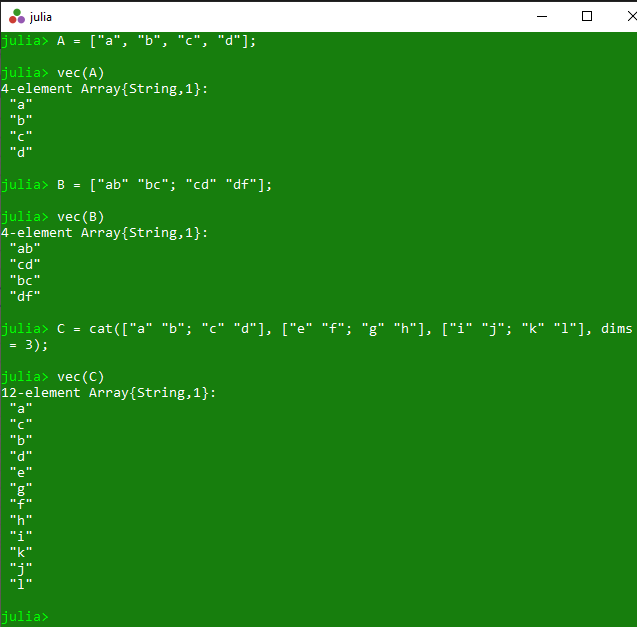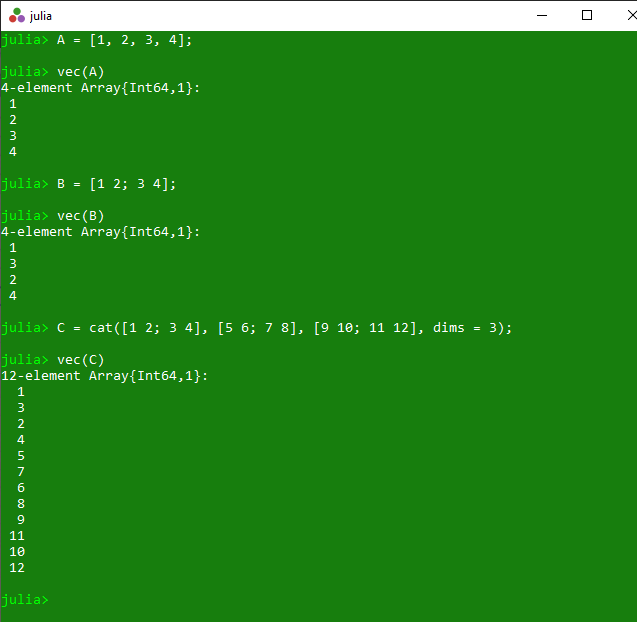Related Articles

# Reshaping array as a vector in Julia – vec() Method

• Last Updated : 25 Mar, 2020

The `vec()` is an inbuilt function in julia which is used to reshape the specified array as a one-dimensional column vector i.e, 1D array.

Syntax:
vec(a::AbstractArray)

Parameters:

• a::AbstractArray: Specified array.

Returns: It returns the reshaped 1D array.

Example 1:

 `# Julia program to illustrate ``# the use of Array vec() method`` ` `# Reshaping the specified array as a ``# one-dimensional column vector ``# i.e, 1D array.``A ``=` `[``"a"``, ``"b"``, ``"c"``, ``"d"``];``println(vec(A))`` ` `# Reshaping the specified array as a ``# one-dimensional column vector ``# i.e, 1D array.``B ``=` `[``"ab"` `"bc"``; ``"cd"` `"df"``];``println(vec(B))`` ` `# Reshaping the specified array as a ``# one-dimensional column vector ``# i.e, 1D array.``C ``=` `cat([``"a"` `"b"``; ``"c"` `"d"``], [``"e"` `"f"``; ``"g"` `"h"``], [``"i"` `"j"``; ``"k"` `"l"``], dims ``=` `3``);``println(vec(C))`

Output:Example 2:

 `# Julia program to illustrate ``# the use of Array vec() method`` ` `# Reshaping the specified array as a ``# one-dimensional column vector ``# i.e, 1D array.``A ``=` `[``1``, ``2``, ``3``, ``4``];``println(vec(A))`` ` `# Reshaping the specified array as a ``# one-dimensional column vector ``# i.e, 1D array.``B ``=` `[``1` `2``; ``3` `4``];``println(vec(B))`` ` `# Reshaping the specified array as a ``# one-dimensional column vector ``# i.e, 1D array.``C ``=` `cat([``1` `2``; ``3` `4``], [``5` `6``; ``7` `8``], [``9` `10``; ``11` `12``], dims ``=` `3``);``println(vec(C))`

Output:My Personal Notes arrow_drop_up